# OCR tables and parse the output¶

In this tutorial, we will illustrate how easily the layoutparser APIs can be used for

1. Recognizing texts in images and store the results with the specified OCR engine

2. Postprocessing of the textual results to create structured data

import layoutparser as lp

import matplotlib.pyplot as plt
%matplotlib inline

import pandas as pd
import numpy as np
import cv2


## Initiate GCV OCR engine and check the image¶

Currently, layoutparser supports two types of OCR engines: Google Cloud Vision and Tesseract OCR engine. And we are going to provide more support in the future. In this toturial, we will use the Google Cloud Vision engine as an example.

ocr_agent = lp.GCVAgent.with_credential("<path/to/your/credential>",
languages = ['en'])


The language_hints tells the GCV which langeuage shall be used for OCRing. For a detailed explanation, please check here.

The example-table is a scan with complicated table structures from https://stacks.cdc.gov/view/cdc/42482/.

image = cv2.imread('data/example-table.jpeg')
plt.imshow(image);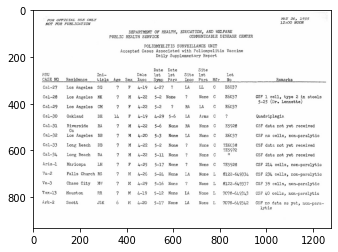## Load images and send for OCR¶

The ocr_agent.detect method can take the image array, or simply the path of the image, for OCR. By default it will return the text in the image, i.e., text = ocr_agent.detect(image).

However, as the layout is complex, the text information is not enough: we would like to directly analyze the response from GCV Engine. We can set the return_response to True. This feature is also supported for other OCR Engines like TesseractOCRAgent.

res = ocr_agent.detect(image, return_response=True)

# Alternative
# res = ocr_agent.detect('data/example-table.jpeg', return_response=True)


## Parse the OCR output and visualize the layout¶

As defined by GCV, there are two different types of output in the response:

1. text_annotations:

In this format, GCV automatically find the best aggregation level for the text, and return the results in a list. We can
use the ocr_agent.gather_text_annotations to reterive this type of information.
2. full_text_annotations

To support better user control, GCV also provides the full_text_annotation output, where it returns the hierarchical structure of the output text. To process this output, we provide the ocr_agent.gather_full_text_annotation function to aggregate the texts of the given aggregation level.

There are 5 levels specified in GCVFeatureType, namely: PAGE, BLOCK, PARA, WORD, SYMBOL.

texts  = ocr_agent.gather_text_annotations(res)
# collect all the texts without coordinates
layout = ocr_agent.gather_full_text_annotation(res, agg_level=lp.GCVFeatureType.WORD)
# collect all the layout elements of the WORD level


And we can use the draw_box or draw_text functions to quickly visualize the detected layout and text information.

These functions are highly customizable. You can change styles of the drawn boxes and texts easily. Please check the documentation for the detailed explanation of the configurable parameters.

As shown below, the draw_text function generates a visualization that:

1. it draws the detected layout with text on the left side and shows the original image on the right canvas for comparison.

2. on the text canvas (left), it also draws a red bounding box for each text region.

lp.draw_text(image, layout, font_size=12, with_box_on_text=True,
text_box_width=1)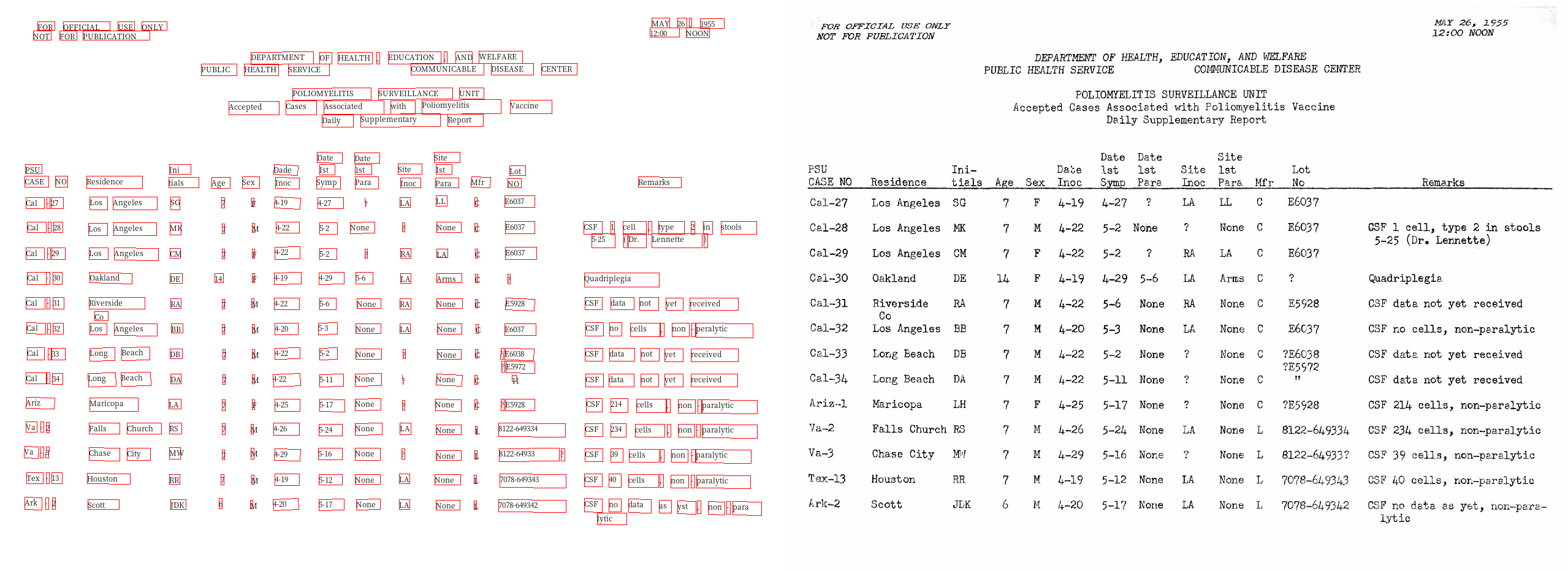## Filter the returned text blocks¶

We find the coordinates of residence column are in the range of $$y\in(300,833)$$ and $$x\in(132, 264)$$. The layout.filter_by function can be used to fetch the texts in the region.

Note: As the OCR engine usually does not provide advanced functions like table detection, the coordinates are found manually by using some image inspecting tools like GIMP.

filtered_residence = layout.filter_by(
lp.Rectangle(x_1=132, y_1=300, x_2=264, y_2=840)
)
lp.draw_text(image, filtered_residence, font_size=16)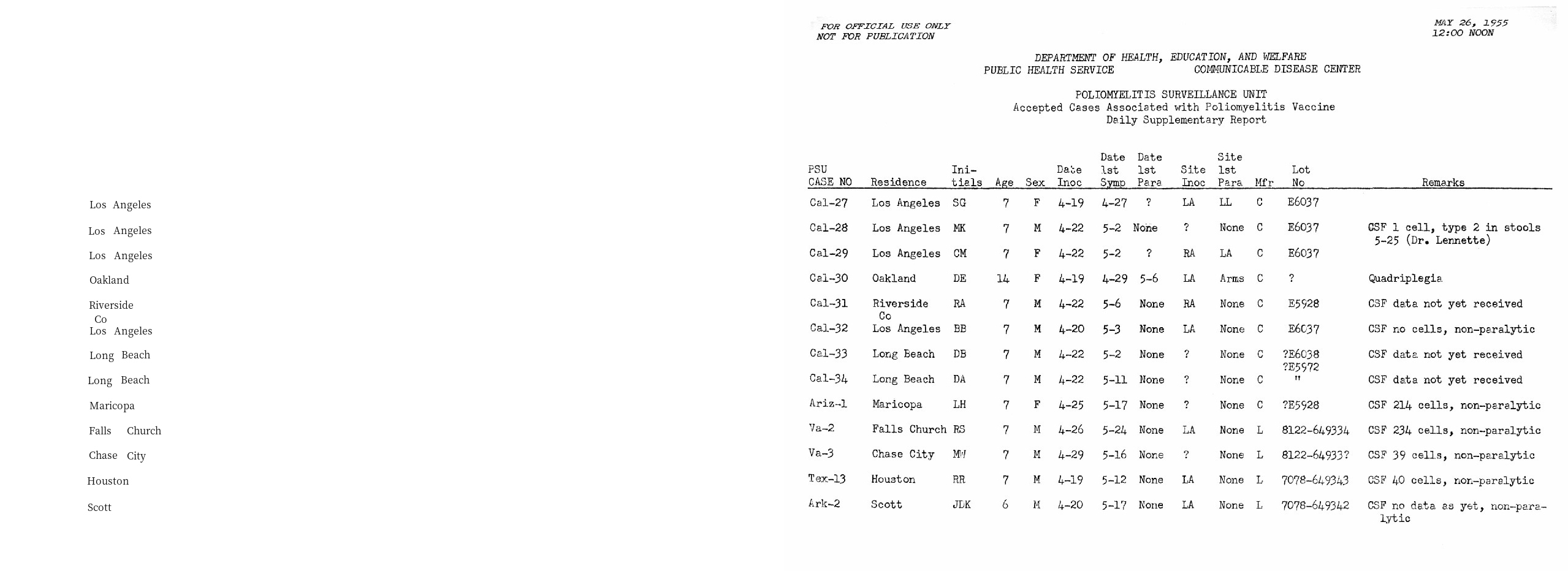And similarily, we can do that for the lot_number column. As sometimes there could be irregularities in the layout as well as the OCR outputs, the layout.filter_by function also supports a soft_margin argument to handle this issue and generate more robust outputs.

filter_lotno = layout.filter_by(
lp.Rectangle(x_1=810, y_1=300, x_2=910, y_2=840),
soft_margin = {"left":10, "right":20} # Without it, the last 4 rows could not be included
)
lp.draw_text(image, filter_lotno, font_size=16)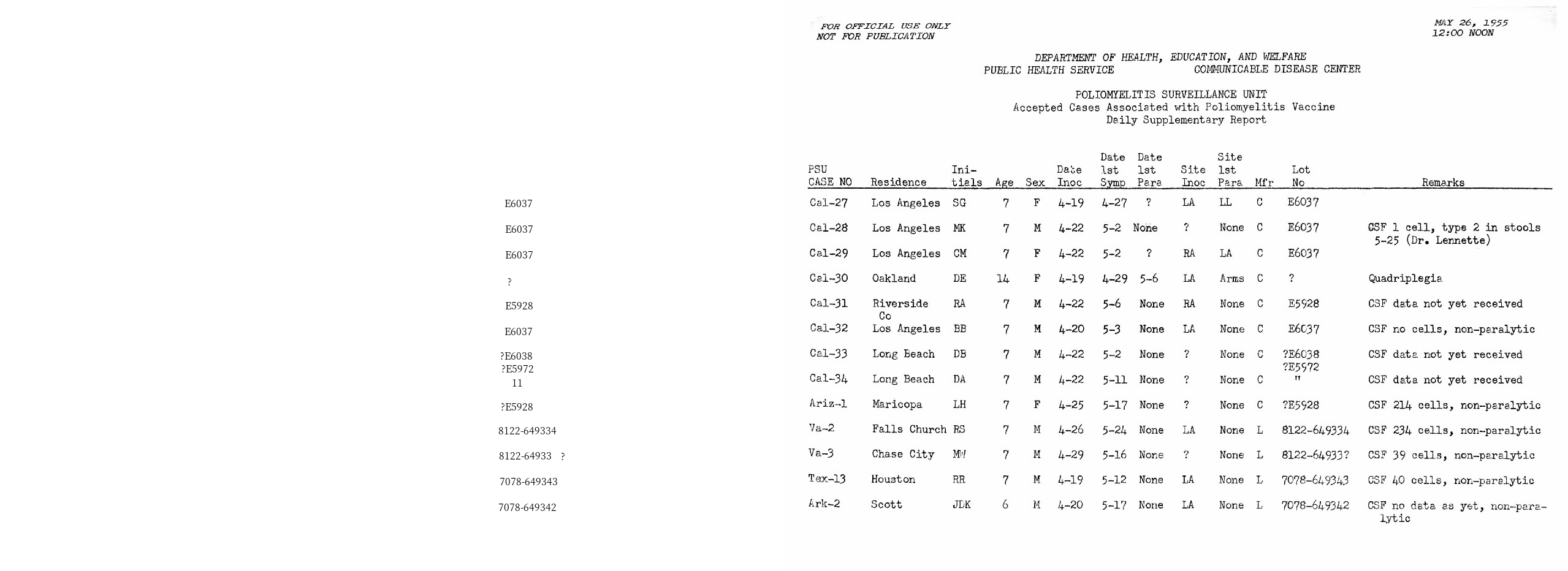### Group Rows based on hard-coded parameteres¶

As there are 13 rows, we can iterate the rows and fetch the row-based information:

y_0 = 307
n_rows = 13
height = 41
y_1 = y_0+n_rows*height

row = []
for y in range(y_0, y_1, height):

interval = lp.Interval(y,y+height, axis='y')
residence_row = filtered_residence.\
filter_by(interval).\
get_texts()

lotno_row = filter_lotno.\
filter_by(interval).\
get_texts()

row.append([''.join(residence_row), ''.join(lotno_row)])

row

[['LosAngeles', 'E6037'],
['LosAngeles', 'E6037'],
['LosAngeles', 'E6037'],
['Oakland', '?'],
['Riverside', 'E5928'],
['LosAngeles', 'E6037'],
['LongBeach', '?E6038'],
['LongBeach', '11'],
['Maricopa', '?E5928'],
['FallsChurch', '8122-649334'],
['ChaseCity', '8122-64933?'],
['Houston', '7078-649343'],
['Scott', '7078-649342']]


### An Alternative Method - Adaptive Grouping lines based on distances¶

blocks = filter_lotno

blocks = sorted(blocks, key = lambda x: x.coordinates)
# Sort the blocks vertically from top to bottom
distances = np.array([b2.coordinates - b1.coordinates for (b1, b2) in zip(blocks, blocks[1:])])
# Calculate the distances:
# y coord for the upper edge of the bottom block -
#   y coord for the bottom edge of the upper block
# And convert to np array for easier post processing
plt.hist(distances, bins=50);
plt.axvline(x=3, color='r');
# Let's have some visualization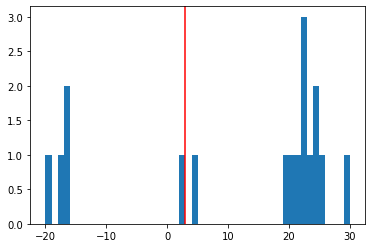According to the distance distribution plot, as well as the OCR results visualization, we can conclude:

• For the negative distances, it’s because there are texts in the same line, e.g., “Los Angeles”

• For the small distances (indicated by the red line in the figure), they are texts in the same table row as the previous one

• For larger distances, they are generated from texts pairs of different rows

distance_th = 0

distances = np.append(, distances) # Append a placeholder for the first word
block_group = (distances>distance_th).cumsum() # Create a block_group based on the distance threshold

block_group

array([ 0,  1,  2,  3,  4,  5,  6,  6,  7,  7,  8,  9,  9, 10, 11, 11, 12,
13])

# Group the blocks by the block_group mask
grouped_blocks = [[] for i in range(max(block_group)+1)]
for i, block in zip(block_group, blocks):
grouped_blocks[i].append(block)


Finally let’s create a function for them

def group_blocks_by_distance(blocks, distance_th):

blocks = sorted(blocks, key = lambda x: x.coordinates)
distances = np.array([b2.coordinates - b1.coordinates for (b1, b2) in zip(blocks, blocks[1:])])

distances = np.append(, distances)
block_group = (distances>distance_th).cumsum()

grouped_blocks = [lp.Layout([]) for i in range(max(block_group)+1)]
for i, block in zip(block_group, blocks):
grouped_blocks[i].append(block)

return grouped_blocks

A = group_blocks_by_distance(filtered_residence, 5)
B = group_blocks_by_distance(filter_lotno, 10)

# And finally we combine the outputs
height_th = 30
idxA, idxB = 0, 0

result = []
while idxA < len(A) and idxB < len(B):
ay = A[idxA].coordinates
by = B[idxB].coordinates
ares, bres = ''.join(A[idxA].get_texts()), ''.join(B[idxB].get_texts())
if abs(ay - by) < height_th:
idxA += 1; idxB += 1
elif ay < by:
idxA += 1; bres = ''
else:
idxB += 1; ares = ''
result.append([ares, bres])

result

[['LosAngeles', 'E6037'],
['AngelesLos', 'E6037'],
['LosAngeles', 'E6037'],
['Oakland', '?'],
['RiversideCoLosAngeles', 'E5928'],
['', 'E6037'],
['BeachLong', '?E6038?E597211'],
['BeachLong', ''],
['Maricopa', '?E5928'],
['FallsChurch', '8122-649334'],
['ChaseCity', '8122-64933?'],
['Houston', '7078-649343'],
['Scott', '7078-649342']]


As we can find, there are mistakes in the 5th and 6h row - Riverside Co and LosAngeles are wrongly combined. This is because the extra row co disrupted the row segmentation algorithm.

## Save the results as a table¶

df = pd.DataFrame(row, columns=['residence', 'lot no'])
df

residence lot no
0 LosAngeles E6037
1 LosAngeles E6037
2 LosAngeles E6037
3 Oakland ?
4 Riverside E5928
5 LosAngeles E6037
6 LongBeach ?E6038
7 LongBeach 11
8 Maricopa ?E5928
9 FallsChurch 8122-649334
10 ChaseCity 8122-64933?
11 Houston 7078-649343
12 Scott 7078-649342
df.to_csv('./data/ocred-example-table.csv', index=None)